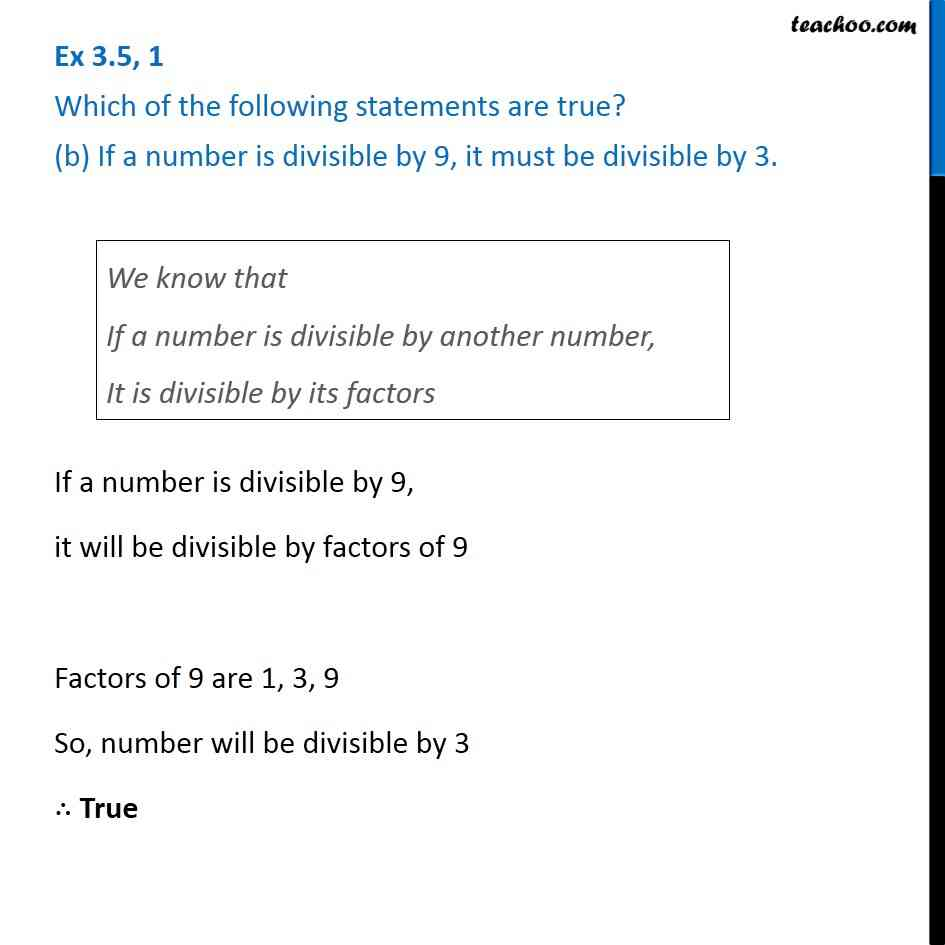Ex 3.5

Chapter 3 Class 6 Playing with Numbers
Serial order wiseLearn in your speed, with individual attention - Teachoo Maths 1-on-1 Class

### Transcript

Ex 3.5, 1 Which of the following statements are true? (b) If a number is divisible by 9, it must be divisible by 3. We know that If a number is divisible by another number, It is divisible by its factors If a number is divisible by 9, it will be divisible by factors of 9 Factors of 9 are 1, 3, 9 So, number will be divisible by 3 ∴ True